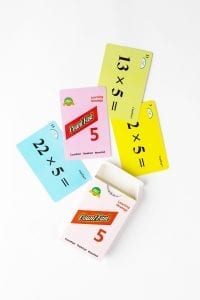# CountFast 5 Home Activities

####OVERVIEW & PURPOSE

Week 4 of the 3rd Grade CountFast program focuses on specific mental strategies for multiplying with the numbers 5, 15, and 50. Spend 15 minutes each day on one of the activities listed in this module.  Card decks should go home with students each day for additional practice with a parent at home. Each week, a new deck is introduced, and the previous deck is for the student to keep at home for continued practice.

#### EDUCATION STANDARDS

1.   CCSS.Math.Content.3.OA.B.5
Apply properties of operations as strategies to multiply and divide.
2.  NCTM Standard: develop a sense of whole numbers and represent and use them in flexible ways, including relating, composing, and decomposing numbers

#### OBJECTIVES

1. Quickly, mentally multiply by 5, 15, or 50.
2. Develop fluency with multiplication calculations.
DAY 1:Use the yellow cards from the deck.  The mental strategy lesson learned today is how to multiply any number by 5.  We can think of 5 as being half of 10.  So it makes sense that we could multiply the original number by 10, and then cut the answer in half.  Practice this strategy with your child and encourage him/her to calculate the answers faster each round, keeping track of his/her time on the time record card.  Your child will be excited every time he/she can get through the cards faster than the time before!DAYS 2 and 3:

Use the same procedure as yesterday.  This time, your child will use the blue cards in the deck to multiply any number by 15.  We learned in class that we can think of 15 as being the same thing as 3 X 5.  To multiply by 15, first multiply the original number by 3.  Then take that answer and multiply it by 5 using the strategy learned yesterday.  For example, 6 X 15 can mentally be solved by first multiplying the 6 times 3 to get 18.  Then take 18 times 10 to get 180, and cut 180 in half to get a final answer of 90.  We will practice this strategy for two days.  Help you child keep track of how long it takes to use the strategy to answer all of the blue cards.  Celebrate each time your child records a faster time.

DAYS 4 and 5:

Use the pink cards in the deck today.  This time, your child will practice multiplying any number by 50.  We learned to think of 50 as being the same thing as 5 X 10.  So, we first multiply the original number by 5 (using the 5’s strategy learned this week), and then taking that answer and multiplying by 10 (by simply adding a zero to the answer).  For example, to multiply 26 by 50, first multiply 26 X 5 (26 X 10 = 260.  260 divided in half is 130.)  Then take the product 130 times 10 by adding a zero.  Final answer is 1300.

#### **Tip**

If your child is not comfortable with the concept of multiplication yet, save these activities for later in the school year, then revisit with greater confidence.

Stay SharpFree flash card decks to keep kids entertained and sharp!

To help you keep your kids math sharp during this crisis, we're giving you 3 free CountFast flash card decks for just \$6 in shipping.  Just have them take the quick quiz for their grade at the link below and you'll get a discount code to use on any three decks.